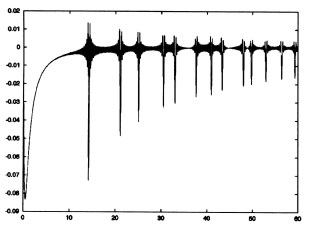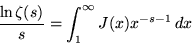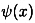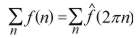## Fourier analysis and number theory[figure by Michael Rubinstein reproduced from Brian Conrey's survey article on the Riemann hypothesis, Notices of the AMS (2003), p. 346]

The above graph illustrates a Fourier-type duality relation between the prime numbers and the zeros of the Riemann zeta function. The function depicted is the Fourier transform of the error term in the Prime Number Theorem, and the visible spikes correspond to the imaginary parts of the zeta zeros.

This Fourier-type duality is most clearly expressed mathematically by the Riemann-Weil explicit formula. What is most interesting, and least understood, about this situation is the fact that the structure of the explicit formula is mirrored by certain dynamical trace formulae.

The first instance of this to be observed involved the Selberg trace formula (discovered in the 1950's) which concerns the geodesic flow on a Riemann surface, relating its periodic orbits and its energy levels, i.e. eigenvalues of the Laplace-Beltrami operator. Here the orbits correspond to the primes and the energy levels to the Riemann zeta zeros. The latter correspondence lends credence to the spectral interpretation of the Riemann zeta function, and the overall situation suggests the existence of some kind of mysterious dynamical system underlying (or "lurking behind" as N. Snaith put it in her Ph.D. thesis) the distribution of prime numbers.

Note that the Selberg trace formula can be thought of as a generalisation of the Poisson summation formula, a very clear instance of Fourier-type duality between functions on a torus.

The wider phenomenon of correspondence between the explicit formulae of number theory (of which the Riemann-Weil formula is just one, important, special case) and dynamical trace formulae points to some fundamental issue of duality which is currently a great mystery, and may turn out to be hugely significant in our understanding of both mathematical and physical reality.

In this page we consider a range of material which involves Fourier analysis in a number theoretical setting.

In H.M. Edwards' excellent book Riemann's Zeta Function (Academic Press, 1974), while discussing in detail the content of Riemann's groundbreaking 1859 paper on the distribution of primes, informs us in Chapter 1 that:

"Riemann was a master of Fourier analysis and his work in developing this theory must certainly be counted among his greatest contributions to mathematics. It is not surprising, therefore, that [in this paper] he immediately applies Fourier inversion to the formula"

Chapter 10 of Edwards' book is called Fourier Analysis and begins:

"One of the basic ideas in Riemann's original paper is, as Chapter 1 shows, the idea of Fourier analysis. This chapter is devoted to the formulation of a more modern approach to Fourier analysis which I believe sheds some light on the meaning of the zeta function and on its relation to the distribution of primes.

The approach to Fourier analysis I have in mind is that in which the fundamental object of study is the algebra of invariant operators on the functions on a group. The group in this instance is the multiplicative groups of positive real numbers..."

At the end of section 10.1:

"The technique by which Riemann derived his formula for [the prime-counting function] J(x) and von Mangoldt his formula for [the logarithmic prime-counting function]is a technique of inversion, of going from the transform to the operator, which might well be called Fourier synthesis - putting the operator back together again when its effect on the invariant subspaces is known."

J.T. Tate, "Fourier analysis in number fields and Hecke's zeta-functions" (1950 Princeton Ph.D. thesis)

"Tate's Thesis", as it is now called, is reproduced as Chapter 15 of Algebraic Number Theory by J.W.S. Cassels and A. Fröhlich (Academic Press, 1967). Unfortunately this appears to be out of print. The introduction explains: "This volume also contains... Tate's doctoral thesis, which is for the first time published here after it had over many years had a deep influence on the subject as a piece of clandestine literature."

Tate proves the functional equations for a very general class of zeta and L-functions by using a version of the Poisson summation formula for idèle groups of algebraic number fields.

B.J. Green's notes on Fourier analysis and the functional equation of the Riemann zeta function

J.-F. Burnol, "On Fourier and zeta(s)"

[Abstract:] "We study some of the interactions between the Fourier Transform and the Riemann zeta function (and Dirichlet-Dedekind-Hecke-Tate L-functions)."

This involves in-depth discussion of such physics-related topics as scattering, causality, the Kramers–Wannier duality relation, and the Hilbert–Pólya idea.

[excerpt]

[Abstract:] "We construct in a Sonine space of entire functions a subspace related to the Riemann zeta function and we show that the quotient contains vectors intrinsically attached to the non-trivial zeros and their multiplicities."

This includes a summary in English. The author has pointed out that the article relates to the 'Hilbert-Pólya idea' for proving the Riemann Hypothesis.

D.C. Brody and C.M. Bender, "Operator-valued zeta functions and Fourier analysis" (preprint 10/2018)

[abstract:] "The Riemann zeta function $\zeta(s)$ is defined as the infinite sum $\sum_{n=1}^{\infty}n^{-s}$, which converges when $\Re s>1$. The Riemann hypothesis asserts that the nontrivial zeros of $\zeta(s)$ lie on the line $\Re s = \frac{1}{2}$. Thus, to find these zeros it is necessary to perform an analytic continuation to a region of complex $s$ for which the defining sum does not converge. This analytic continuation is ordinarily performed by using a functional equation. In this paper it is argued that one can investigate some properties of the Riemann zeta function in the region $\Re s<1$ by allowing operator-valued zeta functions to act on test functions. As an illustration, it is shown that the locations of the trivial zeros can be determined purely from a Fourier series, without relying on an explicit analytic continuation of the functional equation satisfied by $\zeta(s)$."

M. Planat, H. Rosu and S. Perrine, "Ramanujan sums for signal processing of low frequency noise" (submitted to Physical Review E)

[Abstract:] "An aperiodic (low frequency) spectrum may originate from the error term in the mean value of an arithmetical function such as Möbius function or Mangoldt function, which are coding sequences for prime numbers. In the discrete Fourier transform the analyzing wave is periodic and not well suited to represent the low frequency regime. In place we introduce a new signal processing tool based on the Ramanujan sum cq(n), well adapted to the analysis of arithmetical sequences with many resonances p/q. The sums are quasi-periodic versus the time n of the resonance and aperiodic versus the order q of the resonance. New results arise from the use of this Ramanujan-Fourier transform (RFT) in the context of arithmetical and experimental signals."

The final paragraph:

"The other challenge behind Ramanujan sums relates to prime number theory. We just focused our interest to the relation between 1/f noise in communication circuits and the still unproved Riemann hypothesis . The mean value of the modified Mangoldt function b(n), introduced in (29), links Riemann zeros to the 1/f2\alpha noise and to the Möbius function. This should follow from generic properties of the modular group SL(2,Z), the group of 2 by 2 matrices of determinant 1 with integer coefficients , and to the statistical physics of Farey spin chains . See also the link to the theory of Cantorian fractal spacetime ."

 M. Planat, "Noise, oscillators and algebraic randomness", Lecture Notes in Physics 550 (Springer, Berlin, 2000)

 S. Perrine, La theorie de Markoff et ses developpements, ed. T. Ashpool (Chantilly, 2000)

 A. Knauf, "The number theoretical spin chain and the Riemann zeroes", Comm. Math. Phys. 196 (1998), no. 3, 703-731

"It is an empirical observation that the Riemann zeta function can be well approximated in its critical strip using the Number-Theoretical Spin Chain.  A proof of this would imply the Riemann Hypothesis.  Here we relate that question to the one of spectral radii of a family of Markov chains...The general idea is to explain the pseudorandom features of certain number theoretical functions by considering them as observables of a spin chain of statistical mechanics."

 C. Castro and J. Mahecha, "Comments on the Riemann conjecture and index theory on Cantorian fractal space-time", Chaos, Solitons and Fractals 13 (2002) 1407

The paper was inspired by

H. Gopalkrishna Gadiyar and R. Padma, "Ramanujan-Fourier series, the Wiener-Khintchine formula and the distribution of prime pairs", Physica A 269 (1999) 503-510.

[Abstract:] "The Wiener-Khintchine formula plays a central role in statistical mechanics. It is shown that the problem of prime pairs is related to autocorrelation and hence to a Wiener-Khintchine formula. "Experimental" evidence is given for this."

More recently the same authors have produced this:

H. Gopalkrishna Gadiyar and R. Padma, "Rota meets Ramanujan: Probabilistic interpretation of Ramanujan-Fourier series"

[Abstract:] "In this paper the ideas of Rota and Ramanujan are shown to be central to understanding problems in additive number theory. The circle and sieve methods are two different facets of the same theme of interplay between probability and Fourier series used to great advantage by Wiener in engineering."

B.W. Ninham and S. Lidin, "Some remarks on quasi-crystal structure", Acta Crystallographica A 48 (1992) 640-650

[abstract:] "The Fourier transform of skeleton delta function that characterizes the most striking features of experimental quasi-crystal diffraction patterns is evaluated. The result plays a role analogous to the Poisson summation formula for periodic delta funcitons that underlie classical crystallography. The real-space distribution can be interpreted in terms of a backbone comprising a system of intersecting equiangular spirals into which are inscribed (self-similar) gnomons of isosceles triangles with length-to-base ratio the golden mean...In addition to the vertices of these triangles, there is an infinite number of other points that may tile space in two or three dimensions. Other mathematical formulae of relevance are briefly discussed."

[from concluding remarks:] "Perhaps the most interesting feature is that our Fourier-transform sum seems to have much in common with the distribution of the zeros of the Riemann zeta function...! That indicates something of the depth of the problem. That the zeta function ought to come into the scheme of things somehow is not surprising - the Poisson and related summation formulae are special cases of the Jacobi theta function. [Indeed the Bravais lattices can be enumerated systematically through an integral over all possible products and sums of products of any three of the four theta functions in different combinations that automatically preserve translational and rotational symmetries.] The theta-function transformations are themselves just another way of writing the [functional equation of the zeta function]. Additionally, the properties of the zeta function are automatically connected to the theory of prime numbers. So one expects that the Rogers-Ramanujan relations must play a central role in the scheme of things for quasi-crystals."

a proof of the prime number theorem involving Fourier transforms
(summarised by Jonas Wiklund)

N. Wiener's The Fourier Integral and Certain of its Applications, section 17, "The Prime-Number Theorem as a Tauberian Theorem" begins:

"The present section and the three following will be devoted to the application of Tauberian theorems to the problem of the distribution of the primes. The theorem which we shall eventually prove is the famous theorem of Hadamard and de la Vallée Poussin..."

B. van der Pol, "An electro-mechanical investigation of the Riemann zeta function in the critical strip", Bulletin of the AMS 53 (1947)

[commentary from Crandall and Pomerance's Prime Numbers: A Computational Perspective:]

"Peculiar as it may seem today, the scientist and engineer van der Pol did, in the 1940's, exhibit tremendous courage in his 'analog' manifestation of an interesting Fourier decomposition. An integral used by van der Pol was a special case ($\sigma$ = 1/2) of the following relation, valid for $s = \sigma + it, \sigma \in (0,1)$:

$\zeta(s) = s\int_{-\infty}^{\infty} e^{-\sigma\omega}([e^{\omega}]-e^{\omega})e^{-i \omega t} d\omega$

Van der Pol actually built and tested an electronic circuit to carry out the requisite transform in analog fashion for $\sigma=1/2$. In today's primarily digital world it yet remains an open question whether the van der Pol approach can be effectively used with, say, a fast Fourier transform to approximate this interesting integral. In an even more speculative tone, one notes that in principle, at least, there could exist an analog device - say an extremely sophisticated circuit - that sensed the prime numbers, or something about such numbers, in this fashion."

"Beyond the Riemann zeta function and special arithmetic functions that arise in analytic number theory, there are other important entities, the exponential sums. These sums generally contain information - one might say "spectral" information - about special functions and sets of numbers. Thus, exponential sums provide a powerful bridge between complex Fourier analysis and number theory. For a real-valued function, real t, and integers a < b, denote

E(f ;a,b,t) = Sum{a<n<=b} exp(2pi*itf(n))

Each term in such an exponential sum has absolute value 1, but the terms can point in different directions in the complex plane. If the various directions are "random" or "decorrelated" in an appropriate sense, one would expect some cancellation of terms."

R. Crandall and C. Pomerance, Prime Numbers: A Compuational Perspective (Springer, 2001) p. 40.

"The classical periodic orbits are a crucial stepping stone in the understanding of quantum mechanics, in particular when then classical system is chaotic. This situation is very satisfying when one thinks of Poincaré who emphasized the importance of periodic orbits in classical mechanics, but could not have had any idea of what they could mean for quantum mechanics. The set of energy levels and the set of periodic orbits are complementary to each other since they are essentially related through a Fourier transform. Such a relation had been found earlier by the mathematicians in the study of the Laplacian operator on Riemannian surfaces with constant negative curvature. This led to Selberg's trace formula in 1956 which has exactly the same form, but happens to be exact. The mathematical proof, however, is based on the high degree of symmetry of these surfaces which can be compared to the sphere, although the negative curvature allows for many more different shapes."

M.C. Gutzwiller, "Chaos in Quantum Mechanics" (1998 lecture notes)

Unfortunately there are few good references on the Selberg trace formula. Peter Perry suggested the following:

H.P. McKean, "Selberg's trace formula as applied to a compact Riemannian surface", Communications in Pure and Applied Mathematics 25 (1972) 225-246. See also the relevant erratum in Comm. Pure Appl. Math. 27 (1974) p.134.

"This will give a somewhat breezy but in principal complete derivation of Selberg's trace formula for a compact Riemann surface - where the Laplacian has only eigenvalues and no continuous spectrum. There are generalizations to cases where the underlying manifold is non-compact and the Laplacian has continuous spectrum, but these are much more involved analytically.

A useful analogy is Poisson's summation formula for a torus, thought of as a relation between the eigenvalues of the Laplacian (e.g. 4(pi)2 n2 on the circle viewed as [0,1) ) and the lengths of closed geodesics (n for integers n). For a Schwarz class test function the Poisson summation formula says thatand so relates the test function evaluated at lengths on the left to its Fourier transform evaluation at square roots of eigenvalues on the right).

Selberg's trace formula is the spiritual ancestor of the celebrated Duistermaat-Guillemin trace formula. See:

J. Duistermaat and V. Guillemin "The spectrum of positive elliptic operators and periodic bicharacteristics", Invent. Math. 29 (1975), no. 1, 39-79."

S. Albeverio, A. Khrennikov, and R. Cianci, "On the Fourier transform and the spectral properties of the p-adic momentum and Schrodinger operators", Journal of Physics A 30 (1997) 5767-5784.

A. Khrennikov and R. Cianci, "On the Fourier transform and the p-adic momentum and Schrodinger operators", General Relativity and gravitational physics, Rome, 1996 (World Scientific Publications, 1997) 279-285.

N. De Grande-De Kimpe, A. Khrennikov, and L. Van Hamme, "The Fourier transform for p-adic smooth distributions", Lecture Notes in Pure and Applied Mathematics 207 (Dekker, 1999) 97-112.

V. Dimitrov, T. Cooklev and B. Donevsky, "Number theoretic transforms over the golden section quadratic field.", IEEE Trans. Sig. Proc. 43 (1995) 1790-1797

V. Dimitrov,G. Jullien, and W. Miller, "A residue number system implementation of real orthogonal transforms", IEEE Trans. Sig. Proc. 46 (1998) 563-570.

J. Main, V.A. Mandelshtam, G. Wunner and H.S. Taylor, "Riemann zeros and periodic orbit quantization by harmonic inversion"

[abstract:] "In formal analogy with Gutzwiller's semiclassical trace formula, the density of zeros of the Riemann zeta function zeta(z=1/2-iw) can be written as a non-convergent series rho(w)=-pi^{-1} sum_p sum_{m=1}^infty ln(p)p^{-m/2} cos(wm ln(p)) with p running over the prime numbers. We obtain zeros and poles of the zeta function by harmonic inversion of the time signal which is a Fourier transform of rho(w). More than 2500 zeros have been calculated to about 12 digit precision as eigenvalues of small matrices using the method of filter-diagonalization. Due to formal analogy of the zeta function with Gutzwiller's periodic orbit trace formula, the method can be applied to the latter to accurately calculate individual semiclassical eigenenergies and resonance poles for classically chaotic systems. The periodic orbit quantization is demonstrated on the three disk scattering system as a physical example."

[abstract:] "We evaluate the Gutzwiller trace formula for the level density of classically chaotic systems by considering the level density in a bounded energy range and truncating its Fourier integral. This results in a limiting procedure which comprises a convergent semiclassical approximation to a well defined spectral quantity at each stage. We test this result on the spectrum of zeros of the Riemann zeta function, obtaining increasingly good approximations to the level density. The Fourier approach also explains the origin of the convergence problems encountered by the orbit truncation scheme."

Y. Wei, "Dirichlet multiplication and easily-generated function analysis", Computers and Mathematics with Applications 39 (2000) 173-199

[abstract:] "Following sine-cosine functions, sawtooth wave, square wave, triangular wave, and trapezoidal wave become new easily-generated periodic functions. Can a signal be considered to be a superposition of easily-generated functions with different frequencies? In order to answer this question, we generalize Fourier analysis to easily-generated function analysis including easily-generated function series, easily-generated function transformation, and discrete transformation for easily-generated functions. The results in this paper make it possible to represent a signal by use of easily-generated functions. A lot of techniques based on sine-cosine functions can be translated into techniques based on easily-generated functions. Because Dirichlet multiplication in number theory plays a basic role in easily-generated function analysis, we briefly introduce this concept and present a related formula. The main contents of this paper include dirichlet multiplication and a related formula, relations between basic waveforms in electronics, easily-generated function series, easily-generated function transformation, discrete transformation for easily-generated functions, and techniques of easily-generated function analysis."

P. Flajolet, P. Grabner, P. Kirschenhofer, H. Prodinger, and R. Tichy, "Mellin transforms and asymptotics: digital sums", Theoretical Computer Science 123 (1994) 291-314

[abstract:] "Arithmetic functions related to number representation systems exhibit various periodicity phenomena. For instance, a well-known theorem of Delange expresses the total number of ones in the binary representations of the first n integers in terms of a periodic fractal function.

We show that such periodicity phenomena can be analyzed rather systematically using classical tools from analytic number theory, namely the Mellin-Perron formulae. This approach yields naturally the Fourier series involved in the expansions of a variety of digital sums related to number representation systems."

C.F. Woodcock, "Special p-adic analytic functions and Fourier transforms", Journal of Number Theory, 60 (2) (1996) 393-408

archive      tutorial      mystery      new      search      home      contact